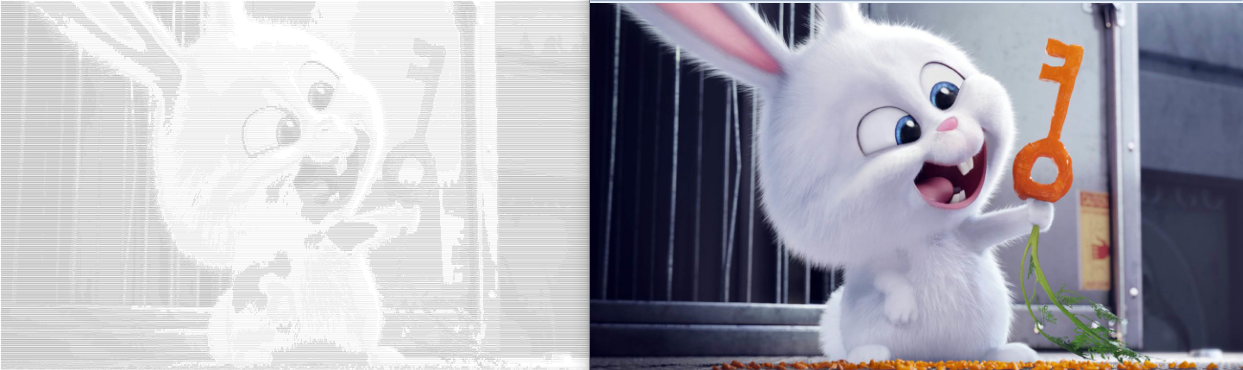# 用PHP实现图片转字符画

1. 图片转字符画的原理就是根据获取到的图像的像素的灰度值，用相应的字符来替换。
2. 总结出了这样一个转化过程：

• 设置像素块大小，将图片都分成这样的像素块，然后选取每个像素块的代表像素
• 获取每个代表像素的RGB值，作为这个像素块的RGB值，计算灰度值
• 根据灰度值选择填充准备好的字符
• 输出
灰度值就是我们看到的黑白图像的一种度量。它可以由公式：`Gray=R*0.3+G*0.59+B*0.11`计算得来。

``````function convert(\$imgName,\$size="medium"){
/*
*    参数说明：
*    imageName    图像名称
*    size        可选参数：low、medium、big 功能：控制输出不同的字符画大小
*    echoText    功能：设置是否保存为txt文件
*/

\$im = getImg(\$imgName);    //自定义函数，获取不同格式的图像资源
\$output="";
\$str='@80GCLft1i;:,. ';    //填充字符

//选取每个像素块的代表点。步长越大，图片越小
switch(\$size){
case "small":
\$stepx=8;
\$stepy=16;
break;
case "medium":
\$stepx=4;
\$stempy=8;
break;
case "big":
\$stepx=2;
\$stepy=4;
break;
default:
\$stepx=4;
\$stempy=8;
break;
}

\$x=imagesx(\$im);
\$y=imagesy(\$im);
for(\$j=0;\$j<\$y;\$j+=\$stepy){
for(\$i=0;\$i<\$x;\$i+=\$stepx){
\$colors=imagecolorsforindex(\$im,imagecolorat(\$im,\$i,\$j));    //获取像素块的代表点RGB信息
\$greyness=(0.3*\$colors["red"]+0.59*\$colors["green"]+0.11*\$colors["blue"])/255;    //灰度值计算公式：Gray=R*0.3+G*0.59+B*0.11
\$offset=(int)ceil(\$greyness*(strlen(\$str)-1));    //根据灰度值选择合适的字符
if(\$offset==(strlen(\$str)-1))
\$output.=" ";    //替换空格为 ；方便网页输出
else
\$output.=\$str[\$offset];
}
\$output.="
";
}

imagedestroy(\$im);
return \$output;
}``````

getImg()是个自定义函数，功能是用来获取不同格式图片的图像资源

``````function getImg(\$imgName){
\$arr=getimagesize(\$imgName);
if(\$arr==1){
return imagecreatefromgif(\$imgName);
}else if(\$arr==2){
return imagecreatefromjpeg(\$imgName);
}else if(\$arr==3){
return imagecreatefrompng(\$imgName);
}else if(...){
...
}
else{
echo "对不起，暂不支持该格式！";
}
}``````

``````\$res=convert("aa.jpg","small",false);
echo "
".\$res."";若要将其保存我文本也很简单，只需做一些简单的替换
\$res=str_replace("
",PHP_EOL,\$res);
\$res=str_replace(" "," ",\$res);